# Age-dependency of PRS for Prostate Cancer

##### Posted on Apr 21, 2023

Recently, PRSs are shown to have relative risks that depend on age, and genetic relative risks decrease with increasing age.

The paper evaluates how the PRS relative risk for prostate cancer depends on age,

• 64274 prostate cancer cases and 46432 controls
• apply a novel weighted proportional hazards model to case-control data to fully utilize age to refine how the relative risk decreased with age.

findings:

• greater relative risks for young men (age 30-55 years) compared with old men (70-88 years) for both relative risk per standard deviation of the PRS and dichotomized according to the upper 90th percentile of the PRS distribution.
• for the largest European ancestral group that could provide reliable resolution, the log-relative risk decreased approximately linearly from age 50 to age 75
• absolute risk predictions differed little from predictions that assumed to a constant relative risk over ages, from short-term to long-term predictions, simplifying implementation of risk discussions into clinical practice.

## Introduction

common diseases increase with age with increasing impact of lifetime exposures, it is critical to evaluate whether the association of a PRS with disease changes with age and the practical implications of ignoring age-dependent risks.

A PRS for an individual is a weighted sum over the doses of selected risk variants, on the order of hundreds to millions of genetic variants, and so creation of a PRS depends on which variants are chosen and how weights are assigned.

The starting point is based on a chosen PRS.

Conti et al.

• men with prostate cancer in the top 10% of the PRS distribution were diagnosed 2.84 years younger than men in the bottom of the distribution
• those with PRS in the top decile of the PRS distribution has an odds ratio of 6.71, compared with PRSs in the 40th-60th percentile, for men aged <= years in contrast to a smaller odds ratio of 4.39 for men older than 55 years.

observation of weakening association of PRS with disease risk as age increases, particularly when many non-genetic risk factors are known to have effects at older ages

the influence of ancestry

• large GWASs are needed to determine the most relevant genetic variants and their weights
• many GWASs have focused on European ancestry.
• the distribution of the PRSs in controls is a normal distribution with mean $\mu$ and variance $\sigma^2$ that both depend on the allele frequencies and the PRS weights

In summary, multiple factors complicate the modeling of the effect of PRSs on prostate cancer risk

• the population distribution of PRSs, which depends on ancestry
• the influence of PRSs on prostate cancer risk, which depends on age
• family history of prostate cancer, which can be confounded with age of diagnosis

The paper refined the analyses reported by Conti et al. by a more extensive evaluation of age, beyond the dichotomy of $\le$ 55 years versus $> 55$ years, and adjusting for the differences in distribution of PRSs across different ancestries.

## Material and Methods

### Age-specific incidence rates for prostate cancer and for death

use age-specific prostate cancer incidence (hazard) rates to create weights for Cox proportional hazards models as well as combine with death hazard rates to compute absolute risks.

Based on life analytic methods, the death hazard rate at age $t$,

$h_t = q_t/(1-q_t/2)\,,$

where $q_t$ is the life table probability of dying between ages $t$ and $t+1$.

### Statistical methods

the association between PRS and age of disease diagnosis were estimated by weighted Cox regression models.

applying Cox regression models to case-control data, using age information, can lead to greater power than logistic regression.

estimate relative risks by use of the Cox model with sampling weights based on population incidence rates to account for how cases and controls were sampled.

• assign weights of $1$ to cases and weights of $1/inc_t$ to controls, where $inc_t$ is the age-specific incidence rate of prostate cancer in a defined ancestry group.
• use survival::survSplit function to fit piece-wise proportional hazards models to allow the relative risks to differ across different age categories,
• as well as the time-transform functions of coxph to model continuous time-dependent coefficients.

The future absolute risk of disease up to age $a_h$, conditional on a man alive and free of disease at age $a_l$ and with a standardized PRS of $z$, depends on

• the baseline population age-specific incidence of disease: $\lambda_0(t)$
• the population age-specific death rate $\delta_0(t)$,
• the log hazard ratio estimated by Cox regression $\beta_t$
$Risk(a_h\mid a_l, z) = \frac{F(a_h\mid z) - F(a_l\mid z)}{S_{disease}(a_l)S_{death}(a_l)}$

where

• $F(a\mid z) = \sum_{t=0}^ae^{\beta_tz}\lambda_0(t)S_{disease}(t\mid z)S_{death}(t)$ is the cumulative probability of disease up to age $a$ accounting for competing risk of death,
• $S_{disease}(a\mid z) = \exp[-\sum_{t=0}^ae^{\beta_tz}\lambda_0(t)]$ is the probability of being free of disease at age $a$
• $S_{death}(a)=\exp[-\sum_{t=0}^a\delta_0(t)]$ is the probability of being alive at age $a$

## Results

• Distribution of PRSs: centered at zero for controls, shifted to greater values for cases.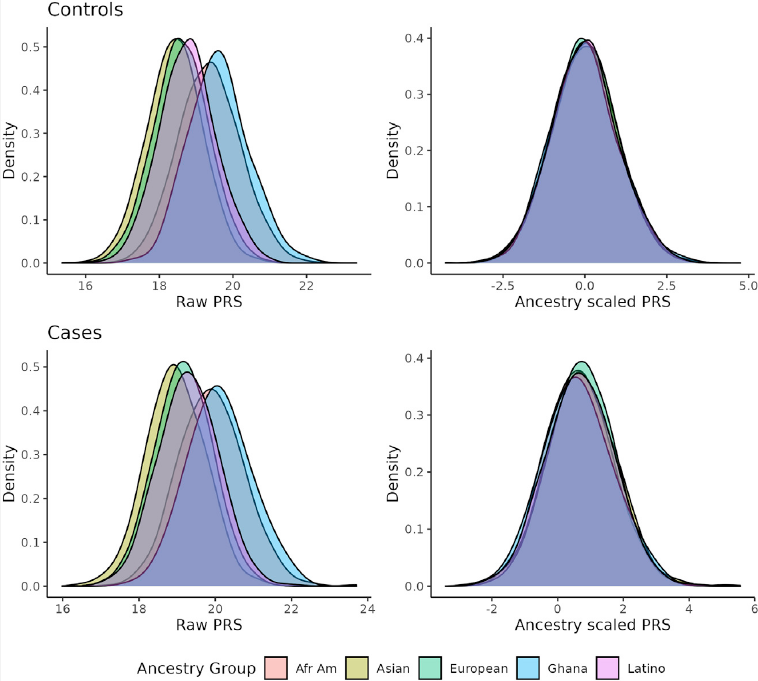• The association of the standardized PRS with age of onset of prostate cancer in the pool of all data, assessed by a weighted Cox proportional hazards model assuming a constant hazard ratio (age of onset is the response ?)
• RR per SD of the PRS
• since the pooled analysis showed a strong departure from a constant relative risk, then perform piece-wise proportional hazards analyses by partitioning age into five age groups and found significant differences in the relative risks across the age groups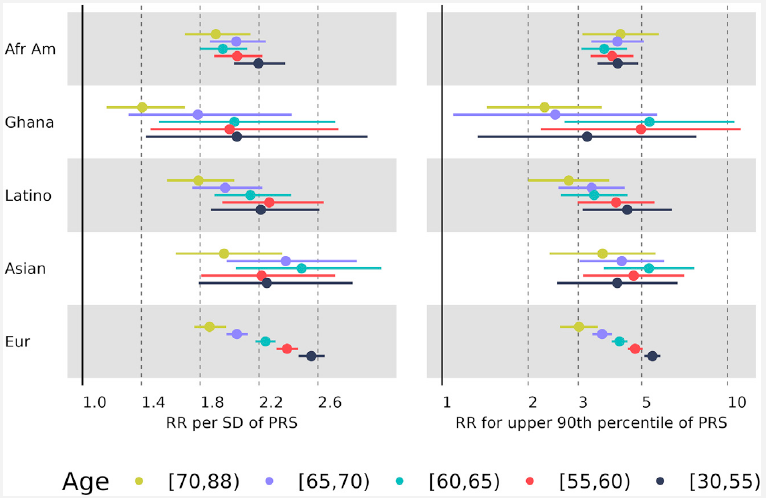• Fit a model with the log-relative risk depending linear on age: $\beta_0 PRS + \beta_1(a-30)PRS$.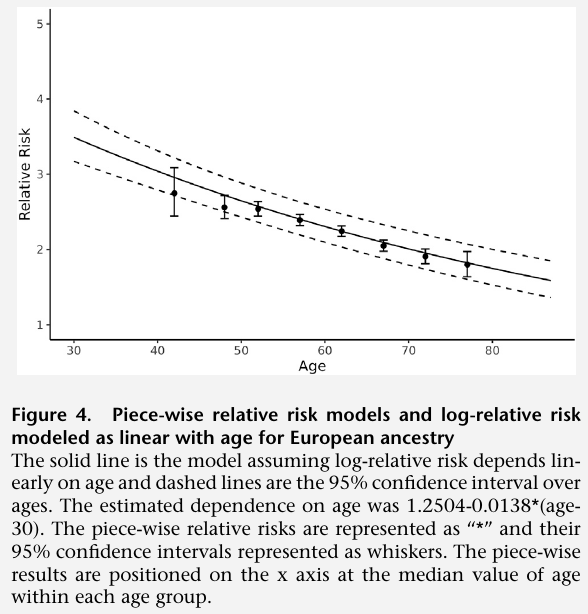Note that the figure does not write $PRS$

• men with a family history of prostate cancer had greater relative risks associated with PRS at all ages compared with men with a negative family history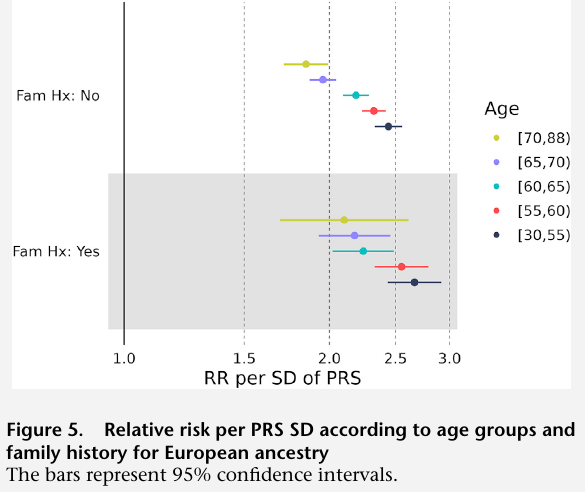• it is important to evaluate how much the decreasing relative risk impacts prediction of future risk when attempting to use PRS for personalized medical recommendations.
• predicting future absolute risk while allowing for decreasing relative risk differed little from predictions that assumed a constant relative risk over ages.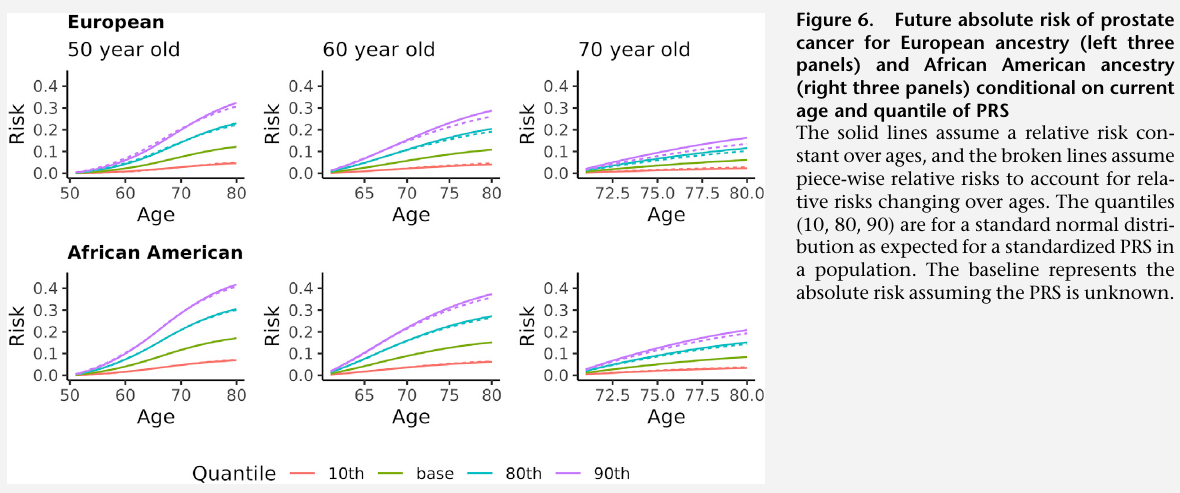Published in categories Note